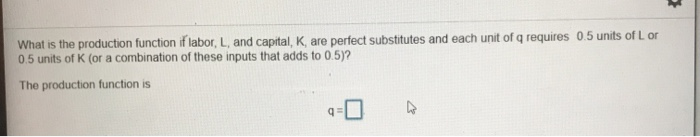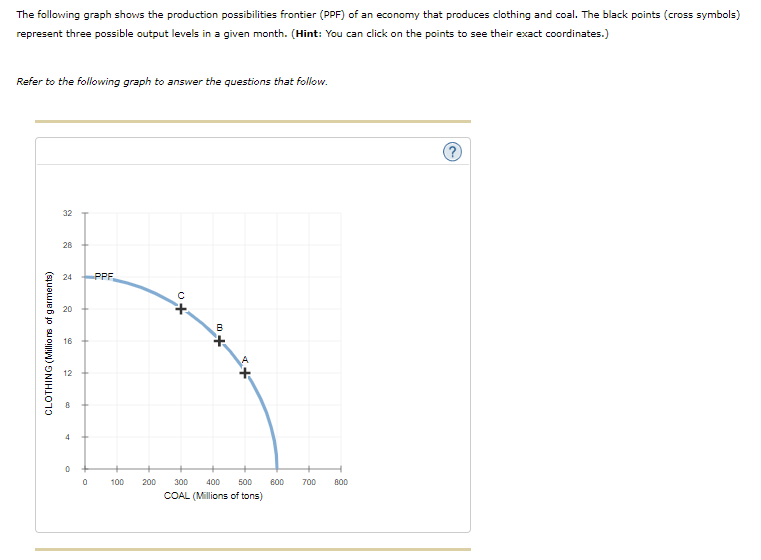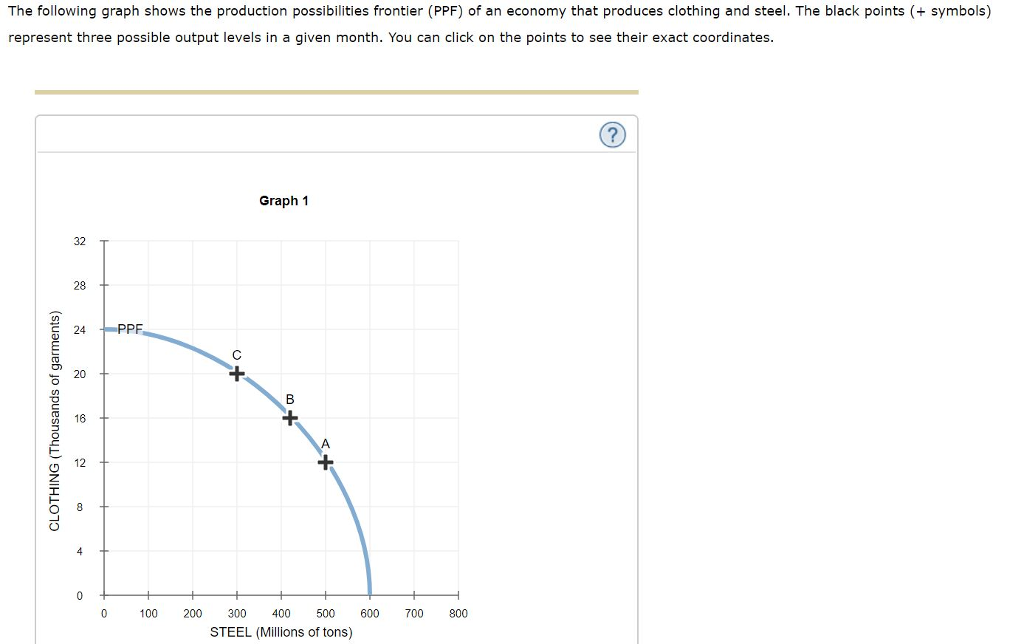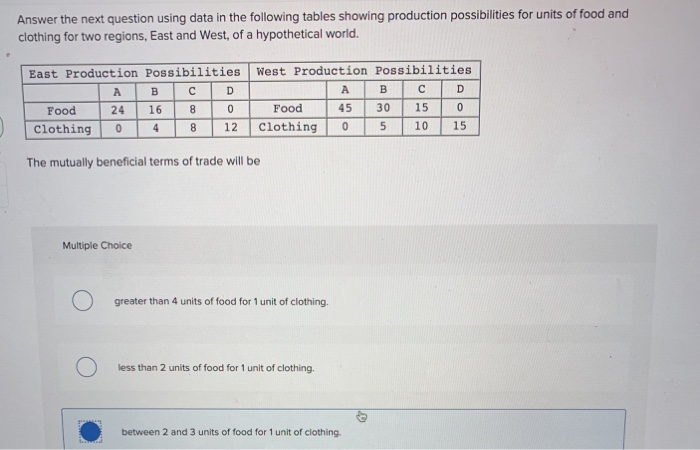Question

Suppose capital and labour are perfect substitutes in production for clothing: 2 units of capital or 2 units of labour produce 1 unit of clothing. Suppose capital and labour are perfect complements in production for food: 1 unit of capital and 1 unit of labour produce 1 unit of food. Suppose the economy has an endowment of 100 units of capital and 200 units of labour. Construct the production possibilities frontier for the economy.(Number the intercepts)

What is the opportunity cost of food in terms of clothing? (Round your answer to two decimal places (e.g., 32.16).)

Opportunity cost:

We need at least 10 more requests to produce the answer.

0 / 10 have requested this problem solution

The more requests, the faster the answer.

All students who have requested the answer will be notified once they are available.

#### Earn Coins

Coins can be redeemed for fabulous gifts.

Similar Homework Help Questions
• ### Suppose capital and labour are perfect substitutes for producing widgets and that it takes 1 unit of capital or 3 units...

Suppose capital and labour are perfect substitutes for producing widgets and that it takes 1 unit of capital or 3 units of labour to produce one widget. If the price of labour is \$4 and the price of capital is \$16, how much labour and capital should a firm use to produce 30 widgets? Explain.

• ### 1. Suppose that, for the production process for quarks, labour and capital are gross complements. Using...

1. Suppose that, for the production process for quarks, labour and capital are gross complements. Using isoquant / isocost analysis, illustrate how the long-run quantity-demanded for labour would be affected by a decrease in the cost of capital. Illustrate both the “substitution effect” and the “scale effect.” 2. Suppose capital and labour are perfect substitutes for producing widgets and that it takes 1 unit of capital or 3 units of labour to produce one widget. If the price of labour...

• ### 4. Consider a production process where capital and labor are perfect complements – two units of...

4. Consider a production process where capital and labor are perfect complements – two units of capital are required for each unit of labor to produce four units of output. 1) Derive the production function for this production process. 2) If the wage rate is \$5 per unit of labor and the rental rate of capital is \$8 per unit of capital, how much capital and labor should the firm employ to minimize the cost of producing 100 units? 3)...

• ### What is the production function if labor, L, and capital, K, are perfect substitutes and each...What is the production function if labor, L, and capital, K, are perfect substitutes and each unit of q requires 0.5 units of Lor 0.5 units of K (or a combination of these inputs that adds to 0.5)? The production function is q=0

• ### Suppose that labor (worker hours) and capital (machine hours) are perfect substitutes in the production of...

Suppose that labor (worker hours) and capital (machine hours) are perfect substitutes in the production of widgets. Each widget can be produced in 20 minutes by a worker or in 15 minutes by a machine. The price of labor is \$20 per hour, and the price of capital is \$30 per hour. Derive the equation for long-run total cost. a. ???? = 60? b. ???? = 170? / 12 c. ???? = 120? d. ???? = 20? / 3 e....

• ### The following graph shows the production possibilities frontier (PPF) of an economy that produces clothing and...The following graph shows the production possibilities frontier (PPF) of an economy that produces clothing and coal. The black points (cross symbols) represent three possible output levels in a given month. (Hint: You can click on the points to see their exact coordinates.) Refer to the following graph to answer the questions that follow. 32 28 24 PPF 20 + B CLOTHING (Millions of garments) 16 + 12 4 0 0 100 200 600 700 800 300 400 500 COAL...

• ### production possibilities

the production possibilities curve below sho the hypothetical relationship between the production of food and clothing in an economy.combiniation food clothingA 0 4b 7 3c 13 2d 18 1e 22 0a) what is the marginal opportunity cost of producing the second unit of clothing?b) what is the total opportunity cost of producing the second uit of closthing?c) what is the marginal opportunity cost of producing the third unit of clothing?e) what is the marginal opportunity cost of producint the thire...

• ### A firm uses labour and capital to produce output according to the production function ??(??, ??) = 4??0.5??0.5, where L...

A firm uses labour and capital to produce output according to the production function ??(??, ??) = 4??0.5??0.5, where L is the number of units of labour and K is the units of capital. The cost of labour is \$40 a unit and the cost of capital is \$10 a unit. a) On a graph, draw an isocost line for this firm, showing combinations of capital and labour that cost \$400 and another isocost line showing combinations that cost \$200....

• ### The following graph shows the production possibilities frontier (PPF) of an economy that produces clothing and...The following graph shows the production possibilities frontier (PPF) of an economy that produces clothing and steel. The black points symbols) represent three possible output levels in a given month. You can click on the points to see their exact coordinates Graph 1 32 28 24 PF 16 100 200 300 400 500 600 700 800 STEEL (Millions of tons) Suppose the economy initially produces 12,000 garments of clothing and 500 million tons of steel, which is represented by point...

• ### Answer the next question using data in the following tables showing production possibilities for units of...Answer the next question using data in the following tables showing production possibilities for units of food and clothing for two regions, East and West, of a hypothetical world. А East Production Possibilities West Production Possibilities A B C D в с Food 24 1680 Food 45 30 15 Clothing 0 4 8 12 Clothing 0 5 10 15 The mutually beneficial terms of trade will be Multiple Choice greater than 4 units of food for 1 unit of clothing...